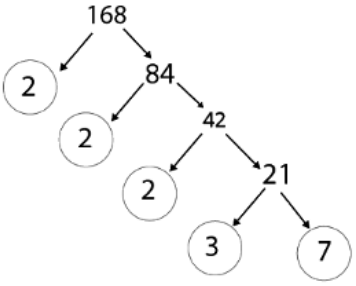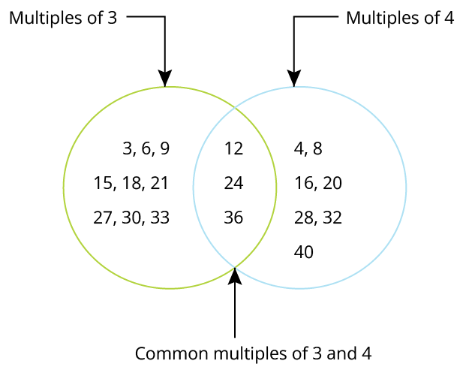Chapter Notes: Be My Multiply

# Chapter Notes: Be My Multiply - Notes | Study Mathematics for Class 5: NCERT - Class 5

 1 Crore+ students have signed up on EduRev. Have you?
What is a Multiple?
• Multiple is a Product of Number and integer.
• Multiples are often called skip counting or counting by numbers.
• Example: 7 x 8 = 56, from this example we can see that 56 is the multiple of 7.
• Example: Let’s look at another example of multiple:
3 x 1 = 3
3 x 2 = 6
3 x 3 = 9
3 x 4 = 12
Here 3, 6, 9, 12 are the multiples of 3.

Point to Remember - 0 is a multiple of every number as the product of 0 multiplied by any number is 0.

What is a Factor?

• To get a product, a number is multiplied and these numbers are factors of the product.
• Example: Let us consider the number 6. 6 can be a product of 1 and 6, and 2 and 3. As a result, the factors of 6 are 1, 2, 3, and 6. Therefore, when finding or solving problems related to factors, only positive numbers, whole numbers, and non-fractional numbers are considered.

Solved Examples

Example 1: What are the Factors of 12?

The factors 12 are: 1, 2, 3, 4, 6, and 12.

Example 2: What are the factors of 36?

The number 36 has many factors other than the one and the number itself, thus the factor of 36 are: 1, 2, 3, 4, 6, 9, 12, 18, and 36.

Factor Tree

• It is a tool used to break down the given number into its prime factors by factorizing the given number.
• We stop this only when we fail to find further factors. Example: Now, let us look at the factor tree of 168.• Step1: Write the pair factors of the given number which on multiplication results in the same number.
• Step2: Mark the prime number as one of the prime factors.
• Step3: Repeat step 1 and step 2 on the quotient (ie. the composite number) until you reach the prime number.
Therefore,168 = 2 x 2 x 2 x 3 x 7
What is a Common Multiple?
• The multiples that are common to two or more numbers are known as the common multiple of those numbers.
• Example: The common multiples of 3 and 4 are:
Multiples of 3: 3, 6, 9, 12, 15, 18, 21, 24, 27, 30, 33, 36.
Multiples of 4: 4, 8, 12, 16, 20, 24, 28, 32, 36, 40.From the multiples of 3 and 4, we can see that 12, 24 and 36 are the common multiple.

Example 1: What are the common multiples of the numbers 10 and 12 by listing multiples?

Before finding the common multiples of 10 and 12, let us find the multiples of both the number:
Multiples of 10:  10, 20, 30, 40, 50, 60, 70, 80, and 90.
Multiples of 12: 12, 24, 36, 48, 60, 72, 84, 96, and 108.
From the above multiple of 10 and 12, we can see that the least common multiple is 60.

What are Common Factors?

• A common factor is a factor that is common to two or more numbers.
• Highest common factor: It is the greatest number which divides the two numbers.
• Lowest common factor: It is the smallest number which divides the two numbers.
• Example: To find the biggest or highest common factor of numbers 20 and 40. Let us firstly write down the factors of 20 and 40.
Factors of 20: 1, 2, 5, 4, 10, and 20.
Factors of 40: 1, 2, 4, 5, 8, 10, 20, and 40.
From the above, it is pretty clear that 20 is the highest common factor of 20 and 40.
The document Chapter Notes: Be My Multiply - Notes | Study Mathematics for Class 5: NCERT - Class 5 is a part of the Class 5 Course Mathematics for Class 5: NCERT.
All you need of Class 5 at this link: Class 5

## Mathematics for Class 5: NCERT

36 videos|73 docs|45 tests
 Use Code STAYHOME200 and get INR 200 additional OFF

## Mathematics for Class 5: NCERT

36 videos|73 docs|45 tests

Track your progress, build streaks, highlight & save important lessons and more!

,

,

,

,

,

,

,

,

,

,

,

,

,

,

,

,

,

,

,

,

,

;